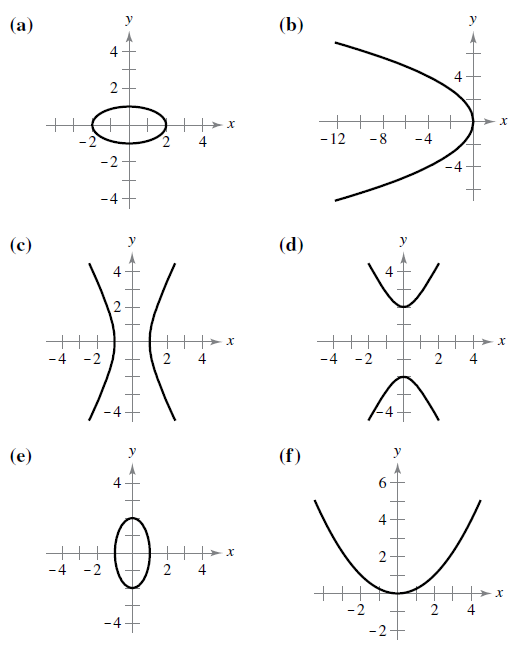Chapter 10, Problem 2RE

Chapter
Section
Textbook Problem

Matching In Exercises 1–6, match the equation with its graph. [The graphs are labeled (a), (b), (c), (d), (e), and (f).]4 x 2 − y 2 = 4

To determine
If the equation 4x2y2=4 matches with its graph when the options are:Explanation

Given:

Explanation:

Consider the given equation: 4x2y2=4

Here, x and y both are squared and one of them has a negative coefficient. Thus, this equation represents a hyperbola.

In order to find whether this hyperbola has a vertical or horizontal axis, represent the given equation in the standard form.

Divide both the sides by 4:

x214y2=1

Rearrange in standard form as:

(x0)212(y0)222</

Still sussing out bartleby?

Check out a sample textbook solution.

See a sample solution

The Solution to Your Study Problems

Bartleby provides explanations to thousands of textbook problems written by our experts, many with advanced degrees!

Get Started

In Exercises 1-4, simplify the expression. x2+6x+8x26x16

Calculus: An Applied Approach (MindTap Course List)

Expand each expression in Exercises 122. x(3x+y)

Finite Mathematics and Applied Calculus (MindTap Course List)

In problems 23-58, perform the indicated operations and simplify. 50.

Mathematical Applications for the Management, Life, and Social Sciences

Finding a Limit In Exercises 17-42, find the limit, if it exists. limx2x(x61)1/3

Calculus: Early Transcendental Functions (MindTap Course List)

Find f(x) if f(x) = 10x2 + cos x. a) 20x sin x + C b) 20x cos x + C c) 103x3cosx+C d) 103x3+sinx+C

Study Guide for Stewart's Single Variable Calculus: Early Transcendentals, 8th

What is the primary factor that determines when it is time to change phases?

Research Methods for the Behavioral Sciences (MindTap Course List)